## f(-5) = -4, and f(5)= 2 linear equation satisfying the conditions , if possible

Question

f(-5) = -4, and f(5)= 2
linear equation satisfying the conditions , if possible

in progress 0
6 months 2021-09-01T16:10:06+00:00 1 Answers 6 views 0

1. Given:

f(-5) = -4, and f(5)= 2.

To find:

The linear equation satisfying the conditions.

Solution:

We have,

f(-5) = -4, and f(5)= 2

It means the function passes through the points (-5,-4) and (5,2). So, the linear equation of the function f is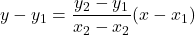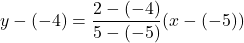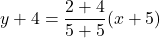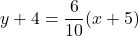On further simplification, we get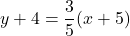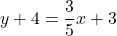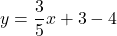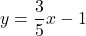Putting y=f(x), we get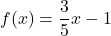Therefore, the required function is.